# Electronics and Communication Engineering - Automatic Control Systems

Exercise : Automatic Control Systems - Section 1
26.
Consider the systems with following open loop transfer functions
1.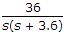2.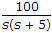3.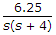If unity feedback is used, the increasing order of time taken for unit step response to settle is
1, 2, 3
3, 1, 2
2, 3, 1
3, 2, 1
Explanation: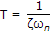. For the three systems the values of ξωn are 1.8, 2.5 and 2 respectively.

Hence values of T are 0.55, 0.4 and 0.5 respectively.

27.
The phase margin and damping ratio have no relation.
True
False
Explanation:

They are related.

28.
For the transport lag G(jω) = e-jωT, the magnitude is always equal to
0
1
10
0.5
Explanation:

Magnitude is 1.

29.
The log magnitude curve for a constant gain K is a
horizontal straight line
horizontal straight line of magnitude 20 log K decibels
an inclined line having slope K
an inclined line having slope -K
Explanation:

The factor K in the numerator of G(jω) gives is straight line at 20 log10 K in Bode plot.

30.
The compensator of the given figure is a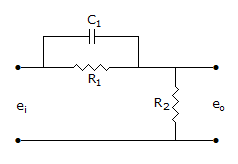lag compensator